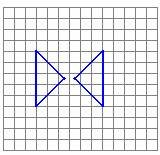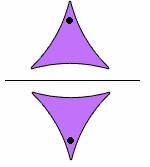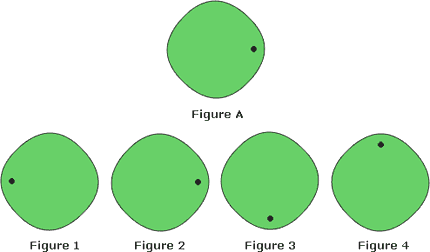## Definition Of Reflection

A Reflection is a transformation in which the figure is the mirror image of the other.

Reflection can also be called as flip.

### Examples of ReflectionIn above figure, one figure is the mirror image of the other. So, the transformation is a reflection.A reflection is the mirror image. Here, the figure on the bottom is the mirror image of the figure on the top.

### Solved Example on Reflection

#### Ques: Which of the figures is a reflection of Figure A?A. Figure 3
B. Figure 2
C. Figure 4
D. Figure 1